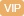| APP | 校园号 | 客服 客服热线：400-863-9889

400-863-988910×7= 40×8= 64+57= 100-24= 600÷3= 64÷2= 312÷3= 120÷4=
【考点】一位数除多位数整数的加法和减法一位数乘多位数【专题】计算题．

 2 5
x=
 3 4
×
 1 2
，利用等式的性，两边同时以
 2 5

【分析】根据比基本性质：两内项之等于外项之可得
 1 4
=5×
 1 5
，再利用式的性两边同时除以
 1 4

 1 6
x=
 2 5
×
 1 2
，再利用等式的性两边同除以
 1 6

【解答】解：：x=
 1 4
 1 5

 1 6
x=
 1 5

 1 6
 1 6
=
 1 5
÷
 1 6

 1 6
 2 5
=
 1 2
：x，

 1 4
=1，
0.3x0.79，

 2 5
 2 5
=
 3 8
÷
 2 5

 2 5
x=
 3 4
×
 1 2

 2 5
x=
 3 8

x4；
x=
 15 16

 x 9
=
 0.7 0.3

=21；
.3x=6.，
x=
 6 5
【点评】此题主要查比的基本性质和等的性的灵应用．

0/0